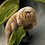# Division of roots

This concerns calculus practice/ limits of sequences and series/ convergence of sequences/ question 4:

Quoting the solution

\begin{align} \lim_{n \to \infty} 2(\sqrt{n+6}\sqrt{n+10}-n) &= 2\lim_{n \to \infty} \frac{(\sqrt{n+6}\sqrt{n+10}-n)(\sqrt{n+6}\sqrt{n+10}+n)}{\sqrt{n+6}\sqrt{n+10}+n} \\ &= 2\lim_{n \to \infty} \frac{(n+6)(n+10)-n^2}{\sqrt{n+6}\sqrt{n+10}+n} \\ &= 2\lim_{n \to \infty} \frac{16n+60}{\sqrt{n+6}\sqrt{n+10}+n} \\ &= 2\lim_{n \to \infty} \frac{16+\frac{60}{n}}{\sqrt{1+\frac{6}{n}}\sqrt{1+\frac{10}{n}}+1} = 16. \end{align}

unquoting

My question is actually only about the last step, where both the nominator and denominator get divided by $\frac{1}{n}$. The guy just pulls $\frac{1}{n}$ into the roots in the denominator. But should it not be, that: $\frac{\sqrt{n+6} \sqrt{n+10}}{n} = \frac{\sqrt{n+6} \sqrt{n+10}}{\sqrt{n^2}} = \sqrt{\frac{n+6}{n^2}}\sqrt{\frac{n+10}{n^2}} = \sqrt{\frac{1}{n} + \frac{6}{n^2}} \sqrt{\frac{1}{n} + \frac{10}{n^2}}$

The difference being that the root expressions converge to 0 instead of 1, and all other parts remaining the same,we have: $2\lim_{n \to \infty} \frac{16+\frac{60}{n}}{\sqrt{ \frac{1}{n}+\frac{6}{n^2}}\sqrt{\frac{1}{n}+\frac{10}{n^2}}+1} = 32$

Can you confirm or am I missing something? Thanks a lot in advance!Note by A Space Bear Smith
2 years, 11 months ago

This discussion board is a place to discuss our Daily Challenges and the math and science related to those challenges. Explanations are more than just a solution — they should explain the steps and thinking strategies that you used to obtain the solution. Comments should further the discussion of math and science.

When posting on Brilliant:

• Use the emojis to react to an explanation, whether you're congratulating a job well done , or just really confused .
• Ask specific questions about the challenge or the steps in somebody's explanation. Well-posed questions can add a lot to the discussion, but posting "I don't understand!" doesn't help anyone.
• Try to contribute something new to the discussion, whether it is an extension, generalization or other idea related to the challenge.

MarkdownAppears as
*italics* or _italics_ italics
**bold** or __bold__ bold
- bulleted- list
• bulleted
• list
1. numbered2. list
1. numbered
2. list
Note: you must add a full line of space before and after lists for them to show up correctly
paragraph 1paragraph 2

paragraph 1

paragraph 2

[example link](https://brilliant.org)example link
> This is a quote
This is a quote
    # I indented these lines
# 4 spaces, and now they show
# up as a code block.

print "hello world"
# I indented these lines
# 4 spaces, and now they show
# up as a code block.

print "hello world"
MathAppears as
Remember to wrap math in $$ ... $$ or $ ... $ to ensure proper formatting.
2 \times 3 $2 \times 3$
2^{34} $2^{34}$
a_{i-1} $a_{i-1}$
\frac{2}{3} $\frac{2}{3}$
\sqrt{2} $\sqrt{2}$
\sum_{i=1}^3 $\sum_{i=1}^3$
\sin \theta $\sin \theta$
\boxed{123} $\boxed{123}$

Sort by:

This is where you made a mistake:

$\cdots = \frac{\sqrt{n+6} \sqrt{n+10}}{\sqrt{n^2}} = \sqrt{\frac{n+6}{n^2}}\sqrt{\frac{n+10}{n^2}} = \cdots$

Note that $\dfrac{a \times b}{c}$ is not equal to $\dfrac ac \times \dfrac bc$.

- 2 years, 11 months ago

Oh man, thanks a bunch! Such an obvious mistake with arithmetic operations . . . . Have a splendid day!

- 2 years, 11 months ago

Yeah no problem.

When dealing with limits (where $n$ becomes unboundedly large), you can (almost) always try to substitute $n=10^6, 10^7, 10^8,\ldots$ and predict what the limit would be.

- 2 years, 11 months ago